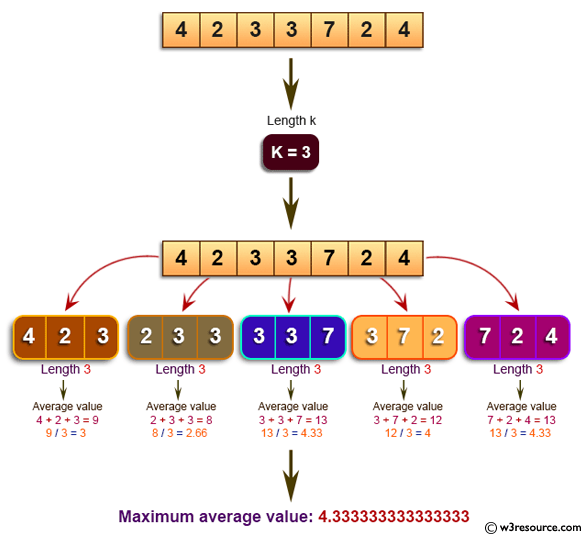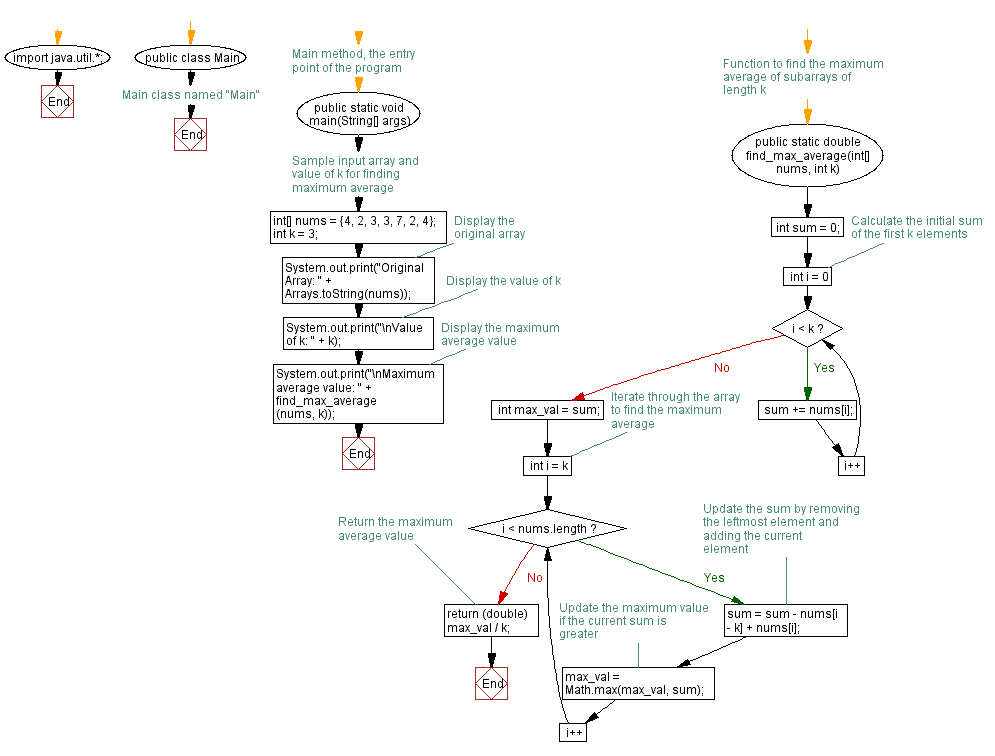# Java Exercises: Find the total number of continuous subarrays in a specified array of integers

## Java Basic: Exercise-203 with Solution

Write a Java program to find the contiguous subarray of given length k which has the maximum average value of a given array of integers. Display the maximum average value.

Example:
Original Array: [4, 2, 3, 3, 7, 2, 4]
Value of k: 3
Maximum average value : 4.333333333333333

Pictorial Presentation:Sample Solution:

Java Code:

``````import java.util.*;
public class Solution {
public static void main(String[] args) {
int[] nums = {4,2,3,3,7,2,4};
int k = 3;
System.out.print("Original Array: "+Arrays.toString(nums));
System.out.print("\nValue of k: "+k);
System.out.print("\nMaximum average value: "+find_max_average(nums, k));
}
public static double find_max_average(int[] nums, int k) {
int sum = 0;
for (int i = 0; i < k; i++) {
sum += nums[i];
}
int max_val = sum;

for (int i = k; i < nums.length; i++) {
sum = sum - nums[i - k] + nums[i];
max_val = Math.max(max_val, sum);
}
return (double) max_val / k;
}
}
```
```

Sample Output:

```Original Array: [4, 2, 3, 3, 7, 2, 4]
Value of k: 3
Maximum average value: 4.333333333333333
```

Flowchart:Java Code Editor:

Company:

What is the difficulty level of this exercise?

﻿

## Java: Tips of the Day

Check if at least two out of three booleans are true:

Rather than writing:

```if (someExpression) {
return true;
} else {
return false;
}
```

Write:

```return someExpression;
```

As for the expression itself, something like this:

```boolean atLeastTwo(boolean a, boolean b, boolean c) {
return a ? (b || c) : (b && c);
}
```

or this (whichever you find easier to grasp):

```boolean atLeastTwo(boolean a, boolean b, boolean c) {
return a && (b || c) || (b && c);
}
```

Ref: https://bit.ly/2OYPQD7